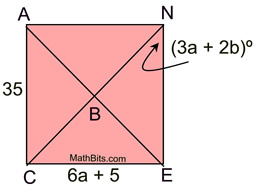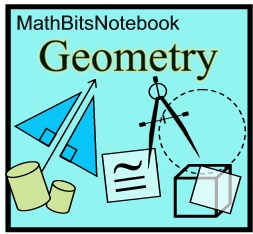Algebraic Practice Problems for Quadrilaterals Terms of Use    Contact Person: Donna RobertsDirections: Read each question carefully. Choose the best answer. State the rules or properties of quadrilaterals that were used to find the answers.

1.
The sides of parallelogram ABCD
are represented as shown.
Find DA.
Choose:
 10 12 24 30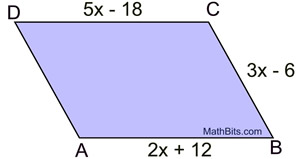2.
In rhombus DOGS, DO = 2p + 9
and OG = 3p - 6.
Find GS.
Choose:
 3 15 19 39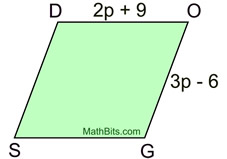3.
The perimeter of quadrilateral ABCD is 46 inches. AB = x + 8,
BC
= 2x + 1, CD = 3x - 6, and DA = 4x - 7.
Find the length of the shortest side of the quadrilateral.
Choose:
 6 inches 9 inches 11 inches 13 inches

4.
Given parallelogram ABCD with
m∠B = 5x and m∠C = 3x+4.
Find the number of degrees in ∠D.
Choose:
 70º 73º 110º 115º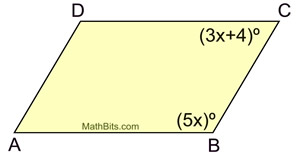5.
The diagonals of rectangle ABCD intersect at E.
AE = x + 4 and CE = 3x - 12.
Find BD.
Choose:
 8 12 16 24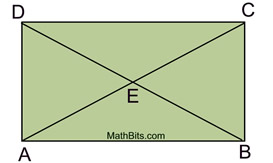6.
In rhombus ABCD, m∠ECB = 5a+4 and m∠EBC = 8a - 5.
Find m∠EBC.
Choose:
 39º 43º 47º 51º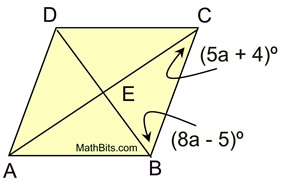7.
Given trapezoid ABCD with median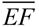(labeled as shown).
Find EF.

Choose:
 8 14 17 19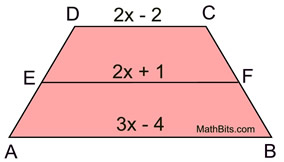8.
In rectangle ABCD, AE = 3x + y,
EC = 2x + y + 7 and DE = 2y + 3x - 1. Find the values of x and y.

Choose:
 x = 7; y = 1 x = 7; y = -1 x = 2; y = 6.5 x = 4; y = -3.5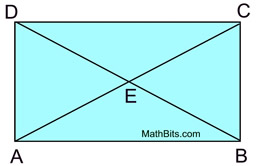9.
Given square ABCD with diagonals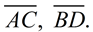If DB = 7x + 1 and AE = 2x + 11, find EB.

Choose:
 7 14 25 50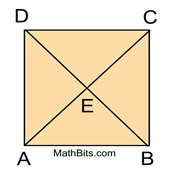10.
Given parallelogram ABCD, labeled as shown.   Find a and b.
Choose:
 a = 10;    b = -30 a = 40;    b = 10 a = 10;    b = 40 a = 40;    b = -10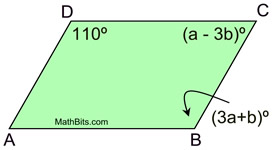11.
Given square ABCD with diagonalsThe m∠DEC = 2a - b and
m∠ABC = a + 2b.   Find a and b.
Choose:
 a = 30;    b = 30 a = 42;    b = 24 a = 54;    b = 18 a = 60;    b = 15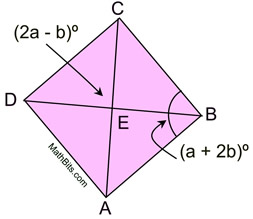12.
Given square CANE with diagonals intersecting at B.   m∠CNE = 3a + 2b,
AC
= 35, and CE = 6a + 5.
Find the value of a + b.
Choose:
 a + b = 15 a + b = 20 a + b = 25 a + b = 45